Courses

# RD Sharma Solutions: Number System- 1 Class 9 Notes | EduRev

## Class 9 : RD Sharma Solutions: Number System- 1 Class 9 Notes | EduRev

The document RD Sharma Solutions: Number System- 1 Class 9 Notes | EduRev is a part of the Class 9 Course Mathematics (Maths) Class 9.
All you need of Class 9 at this link: Class 9

RD Sharma Solutions: Exercise 1.1 - Number System

Q.1. Is zero a rational number? Can you write it in the form p/q, where p and q are integers and q ≠ 0?

Proof: Yes, zero is a rational number because it is either terminating or non-terminating so we can write in the form of p/q, where p and q are natural numbers and q is not equal to zero.

So,

p = 0,q = 1,2,3...

Therefore,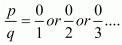Q.2. Find five rational numbers between 1 and 2.

Proof: We need to find 5 rational numbers between 1 and 2.

Consider,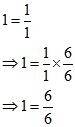And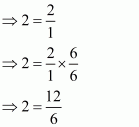So, five rational numbers between 6/6 and 12/6  will be 7/6, 8/6, 9/6, 10/6, 11/6.

Hence 5 rational numbers between 1 and 2 are: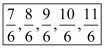OR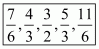Q.3. Find six rational numbers between 3 and 4.

Proof: We need to find 6 rational numbers between 3 and 4.

Consider,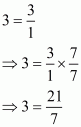And,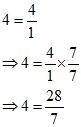So, six rational numbers between 21/7 and 28/7 will be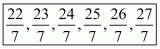Hence 6 rational numbers between 3 and 4 areQ.4. Find five rational numbers between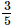and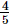Proof: We need to find 5 rational numbers betweenandSince, LCM of denominators= LCM (5,5) = 5

So, consider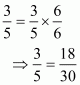And,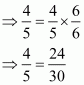Hence 5 rational numbers betweenandare: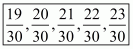OR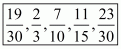Q.5. Are the following statements true or false? Give reasons for your answer.

(i) Every whole number is a natural number.

(ii) Every integer is a rational number.

(iii) Every rational number is an integer.

(iv) Every natural number is a whole number.

(v) Every integer is a whole number.

(vi) Every rational number is a whole number.

Proof: (i) False, because whole numbers start from zero and natural numbers start from one

(ii) True, because it can be written in the form of a fraction with denominator 1

(iii) False, rational numbers are represented in the form of fractions. Integers can be represented in the form of fractions but all fractions are not integers. for example: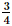is a rational number but not an integer.

(iv) True, because natural numbers belong to whole numbers

(v) False, because set of whole numbers contains only zero and set of positive integers, whereas set of integers is the collection of zero and all positive and negative integers.

(vi) False, because rational numbers include fractions but set of whole number does not include fractions

Offer running on EduRev: Apply code STAYHOME200 to get INR 200 off on our premium plan EduRev Infinity!

## Mathematics (Maths) Class 9

46 videos|323 docs|108 tests

,

,

,

,

,

,

,

,

,

,

,

,

,

,

,

,

,

,

,

,

,

;